# sequence to be detected 1100. Choose either JK flipflop or D flipflop Lab 3: State Machine...

sequence to be detected 1100. Choose either JK flipflop or D flipflopLab 3: State Machine Sequence Detector Introduction This lab will build on what you have learnt so far in this module on the topic of sequential circuit analysis and design. In the previous lab you have investigated combination logic. Today, we will focus on designing a sequential circuit for a sequence detector using edge-triggered D- type or JK-type flip-flops. We will use the concept of a finite state machine to design a circuit capable of detecting a specific pattern of bits in an arbitrary length serial bit sequence, as discussed in class. Design Specification You are required to work in pairs to design a 4-bit sequence detector which analyses an incoming serial data stream, and outputs a logic HIGH, for one clock cycle, when a specific sequence is detected. Overlapping sequences should not produce a logic HIGH output in this design exercise. The sequence to be detected is determined by the last two digits of the lead partner's student number, according to the table below the lead partner must be decided between you, and should be indicated on the report sheet provided by ticking the appropriate box). Sequence to be detected 0101 Second last digit even number even number odd number Last digit even number odd number even number odd number 0100 1100 odd number 1011 All sequences are left-hand started i.e. the leftmost number enters the sequence detector first. The detector must be able to detect the sequence above from every possible input stream that is contained within, and must not give any false positives. As an example sequence, try inputting: 01011 01110 01101 01010 01000. This contains all the 4-bit sequences tabulated above, so should give a logic HIGH output for one clock cycle on the last bit of the relevant sequence. Design Procedure and Report You are required to carry out your design using one of the following flip-flop types: a) JK flip-flops, or b) D flip-flops. There are two kinds of state machines: Moore machines and Mealy machines. In Moore machines, the output depends only on the current state stored inside the machine. In Mealy machines, the output depends on both the stored state and the current input. You need to decide which machine you choose to design for this detector. Or you may design both, compare them and explain the difference between them.

This Homework Help Question: "sequence to be detected 1100. Choose either JK flipflop or D flipflop Lab 3: State Machine..." No answers yet.

We need 10 more requests to produce the answer to this homework help question. Share with your friends to get the answer faster!

0 /10 have requested the answer to this homework help question.

Once 10 people have made a request, the answer to this question will be available in 1-2 days.
All students who have requested the answer will be notified once they are available.
##### Add Answer of: sequence to be detected 1100. Choose either JK flipflop or D flipflop Lab 3: State Machine...
Similar Homework Help Questions
• ### Design a 4-bit serial bit sequence detector. The input to your state detector is called DIN...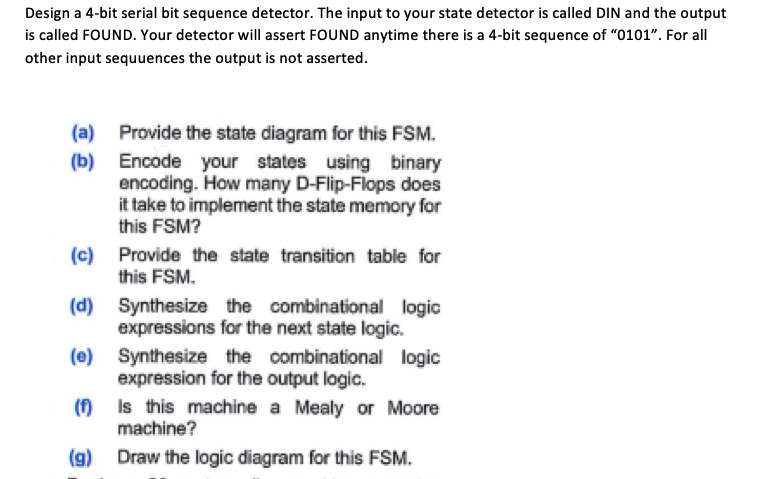Design a 4-bit serial bit sequence detector. The input to your state detector is called DIN and the output is called FOUND. Your detector will assert FOUND anytime there is a 4-bit sequence of "0101". For all other input sequuences the output is not asserted. (a) (b) Provide the state diagram for this FSM. Encode your states using binary encoding. How many D-Flip-Flops does it take to implement the state memory for this FSM? (c) Provide the state transition table...

• ### 4. [10 Pts] Design a sequential circuit for a sequence detector that detects the sequence 10011.A continuous bit stream is fed at the input of the circuit. Every time the circuit detects the sequ...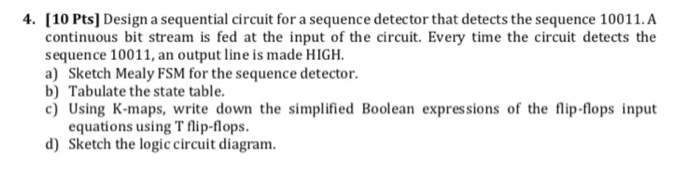4. [10 Pts] Design a sequential circuit for a sequence detector that detects the sequence 10011.A continuous bit stream is fed at the input of the circuit. Every time the circuit detects the sequence 10011, an output line is made HIGH a) Sketch Mealy FSM for the sequence detector. b) Tabulate the state table c) Using K-maps, write down the simplified Boolean expressions of the flip-flops input equations using T flip-flops d) Sketch the logic circuit diagram 4. [10 Pts]...

• ### Design a sequential circuit for a single-input and single output Moore-type FSM that produces an ...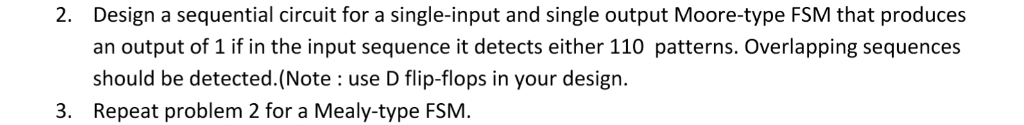digital logic Design a sequential circuit for a single-input and single output Moore-type FSM that produces an output of 1 if in the input sequence it detects either 110 patterns. Overlapping sequences should be detected.(Note : use D flip-flops in your design. Repeat problem 2 for a Mealy-type FSM 2. 3. Design a sequential circuit for a single-input and single output Moore-type FSM that produces an output of 1 if in the input sequence it detects either 110 patterns. Overlapping...

• ### Can anyone explain how can you get the above logic diagram? I have no clue how the answer is like that. I've been trying to derive the truth table and draw the logic diagram, but it's not the...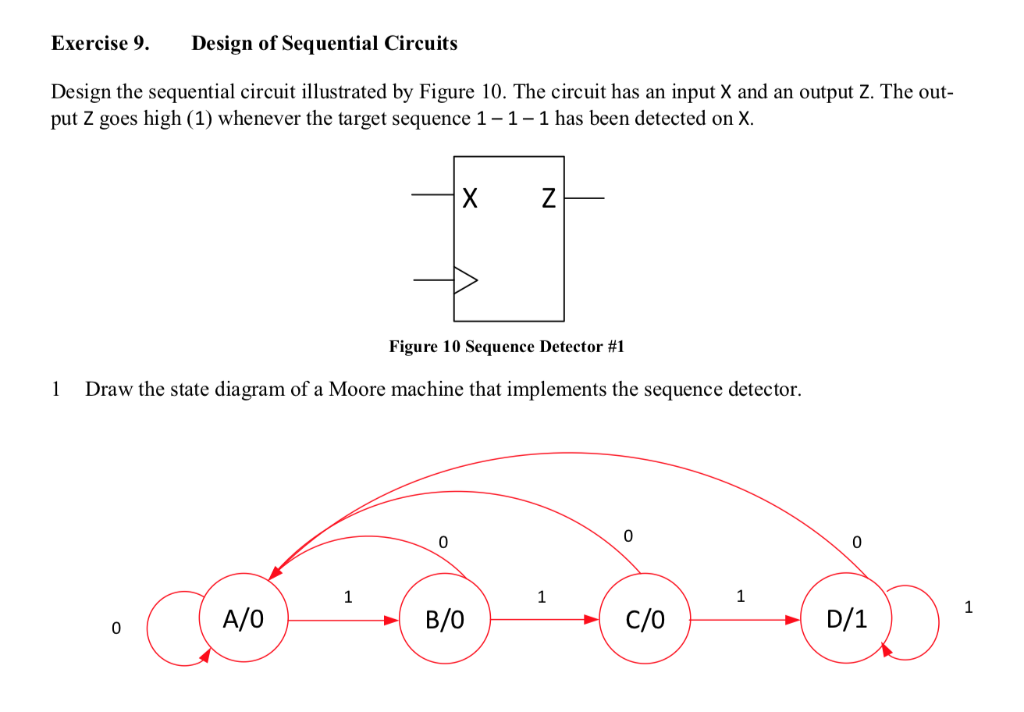Can anyone explain how can you get the above logic diagram? I have no clue how the answer is like that. I've been trying to derive the truth table and draw the logic diagram, but it's not the same as the above answer. Exercise 9. Design of Sequential Circuits Design the sequential circuit illustrated by Figure 10. The circuit has an input X and an output Z. The out put Z goes high (1) whenever the target sequence 1-1-1 has...

• ### please provide the answers of the 4 points thanks? C Tarek Ould-Bachir, PEng,PhD. Design of Sequential Circuits ise 10. nesign the sequential circuit illustrated by Figure 11 Sequence Detector. Th...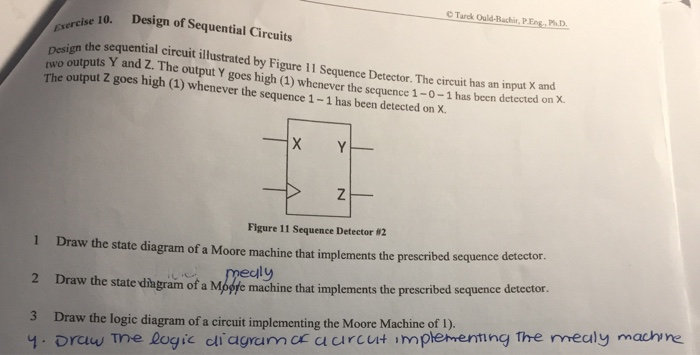please provide the answers of the 4 points thanks? C Tarek Ould-Bachir, PEng,PhD. Design of Sequential Circuits ise 10. nesign the sequential circuit illustrated by Figure 11 Sequence Detector. The cireuit has an input X and wo outputs Y and Z. The output Y goes high (1) whenever the sequence 1-0-1 has been detected on x. The output Z goes high (1) whenever the sequence 1-1 has been detected on X. Figure 11 Sequence Detector #2 1 Draw the state...

• ### 1. Given the state diagram shown below for a two-state synchronous sequential Mealy circuit with input....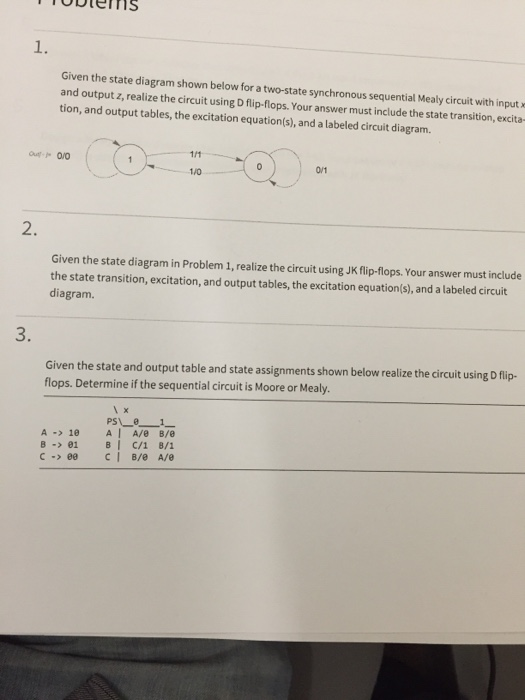1. Given the state diagram shown below for a two-state synchronous sequential Mealy circuit with input. and output z, realize the circuit using D flip-flops. Your answer must include the state transition,excita- tion, and output tables, the excitation equation(s), and a labeled circuit diagram 1/0 2. Given the state diagram in Problem 1, realize the circuit using JK flip-flops. Your answer must include the state transition, excitation, and output tables, the excitation equation(s), and a labeled circuit diagram. 3. Given...

• ### Design a sequential circuit whose output Z becomes 1 when the pattern "01101" is found at 1-bit input X under the following conditions. (1) Use a D flip-flop for the flip-flop used as a Mealy...

Design a sequential circuit whose output Z becomes 1 when the pattern "01101" is found at 1-bit input X under the following conditions. (1) Use a D flip-flop for the flip-flop used as a Mealy machine (2) Use a RS flip-flop for the flip-flop used as a Moore machine

• ### DIGITAL ELECTRONICSQ. 1 Design the sequential circuit whose state table is given below using D-Flip and combinational gates.                  Q. 2 A sequence recognizer is to be designed to detect an input sequence of ‘1011’. The Mealy model state diagram is as shown below. The sequence recognizer outputs a ‘1’ on the detection of this input sequence. The sequential circuit is to be designed using JK and D type flip-flops.            ...

• ### Finite State Machine Design

(Finite State Machine Design) Repeat Problem 1 using the state assignment Y1Y0: A = 00, B=11, C=01, D=10.1. (Flip-Flops) The characteristic equations for a D flip-flop determine that the output Q is updated to either 0 or 1 after each clock cycle. A loadable Dflip-flop has one additional input Load that allows data to be stored in the flip-flop for more than a single clock cycle. Design a loadable D flip-flop with anactive low input Load using a normal D...

• ### 1. A sequential circuit has one JK flip-flop A, one input x, and one output y....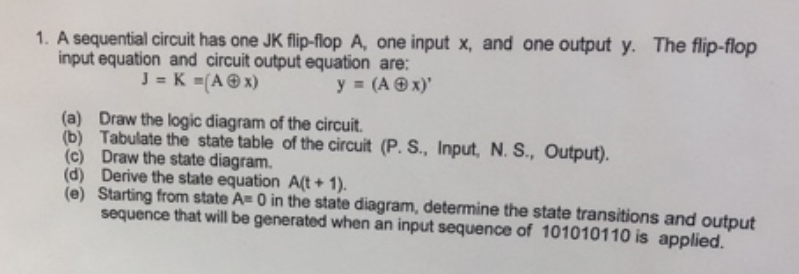1. A sequential circuit has one JK flip-flop A, one input x, and one output y. The flip-flop input equation and circuit output equation are: (a) Draw the logic diagram of the circuit (b) Tabulate the state table of the circuit (P. S., Input, N. S., Output). (c) Draw the state diagram. (d) Derive the state equation A(t+ 1). (e) Starting from state A 0 in the state diagram, determine the state transitions and output sequence that will be generated...

Free Homework App